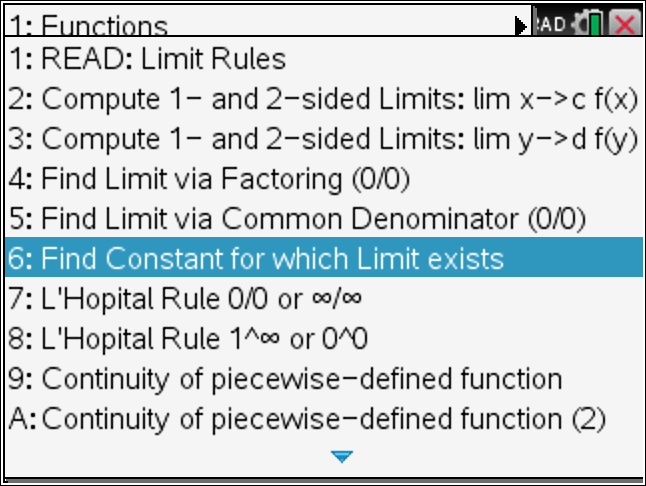## Z-Transforms using the TiNspire CX CAS

We again listened to your request and added Z – Transforms and Inverse Z-Transforms to the Differential Equation Made Easy APP at www.TiNspireApps.com .

Lets take a look at the menu to see the lengthy listing of the forward Z Transforms.

Here is an example of the Z-Transform of an exponential function:

Below find the menu options for the Inverse Z-Transform:

Options A and B offer a Table of Z Transforms and Partial Fraction Decomposition which are useful tools when doing Z Transforms.

Posted on Categories transformTags ,

## TiNspire: CoFactors , Determinant , A as Product of Elementary Matrices

To Find a Determinant given CoFactors in a Row use Linear Algebra Made Easy at www.TiNspireApps.com and enter your quadratic Matrix and selected Row as follows:

When selecting Row2 we get the following CoFactors and of course the same determinant:

To Express a quadratic Matrix as a Product of Elementary Matrices we simply keep track of the RREF Operations when finding its Inverse Matrix. See below:

and finally

Voila !

Posted on Categories linear algebra

## TiNspire CX CAS : Solving 1. order Differential Equations – Step by Step

If we are asked to solve the 1. Order Differential Equation y’+4y=8 we see that it is Linear in y and thus use option B in Differential Equations Made Easy at www.TiNspireApps.com as shown below:

Next enter the coefficients 4 and 8 and leave the 2. box empty since we are not given any initial conditions.

To get the general solution :

If we are given this first order homogeneous Differential Equation :

dy/dx + 5yx^3 = 0 we think of it as dy/dx = – 5yx^3 which calls for Separable Differential Equations which are solved using option 3 as follows :

Here we add an initial condition which allows to find a particular solution:

and finally the separation of variables method yields the particular solution:

## TiNspire CX CAS : Integration by Parts – Step by Step

To perform Integration by Parts just enter the given functions under Step by Step Integration using Calculus Made Easy at www.TinspireApps.com as shown below

Then enter given function and view steps in bottom box

And further:

Another example:

## TiNspire CX : Euler Method (Differential Equations)

When solving a Differential Equation y’=y*(5-y) , y(0)=9 numerically using the Euler Method given stepsize of 0.1 use the Differential Equations made Easy app at www.tinspireapps.com and select Euler Method in the Menu as shown below :

Now you just enter the Differential Equation in the top box and the starting point and the step size in the bottom box as shown below:

The bottom box now shows the step by step solution of the Euler Method. Works correctly for any given Differential Equation.

Alternative to the Euler Method you may also the built-in Runga Kutta RK4 method.

## TiNspire CX Limit Solver

Use belows Limit Solver when asked to find a constant for which a limit exists. Ex: What is a in (x^2+x-a) /(x-2) as x approaches 2?

To find the constant a for which the limit exists using the TiNspire CX CAS use Calculus Made Easy at www.TinspireApps.com , select option 6 in the Limits menu :When entering the above example, we find the Limit to be 5 when the constant a=6 .

## TiNspire CX CAS: Nonhomogeneous, Nonlinear Cauchy Euler 3. order Differential Equation

To solve a 3. order Cauchy Euler Differential that is Nonhomogeneous and NonLinear you would use the Differential Equations Made Easy at www.TiNspireApps.com and enter the coefficients of the Differential Equations as follows:

As can be seen, the substitution y=x^n allows us to find the zeros of the homogeneous Differential Equation and its solution below. Now, we are after the nonhomogenous solution which involves find the 4 Wronskians W, W1, W2, W3 using the Variation of Parameter method:

After finding the 3 v_i, their integration allows us to find the final solution

Puuh, that was a lot of work…If you want to skip watch all the steps you might just jump straight to the final solution.

## TiNSpire CX: Solve System of Differential Equations using LaPlace Transform – Step by Step

Say you have to solve the system of Differential Equations shown in below’s image. Launch the Differential Equations Made Easy app (download at www.TiNspireApps.com) , go to Laplace Transforms in the menu and just type in as shown below:

Scrolling down to view all steps finally shows the correct final answer:

Posted on Categories differential equation, laplace transform

## Solving Fractions and Quadratics using the TiNSpire – Step by Step

Say your teacher has some fancy fractions to solve for you and you have a volleyball game , play practice and to prepare for the SAT next Saturday. So you take out your TiNspire CX CAS, launch the STEP BY STEP EQUATION SOLVER app from www.TiNspireApps.com and get a quick lesson on how to solve those fractions…which turns out not too difficult after following the provided steps below:

We select option 5 :

1. problem:

to get 5/14 . The trick is to multiply the given fractions by the product of their denominators (bottoms) to get a much easier equation to solve.

Here is another one:

and

It always works!

Quadratic equations can also be solved step by step. Here is one:

and

Even equations containing only variables can be solved (for x):

Posted on Categories algebra, tinspirecx